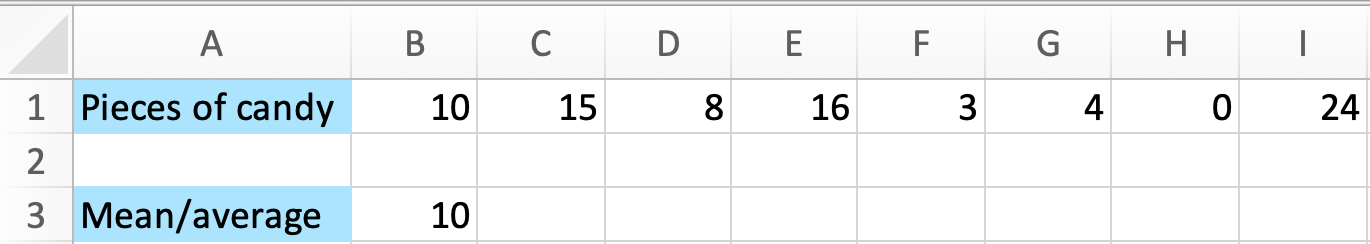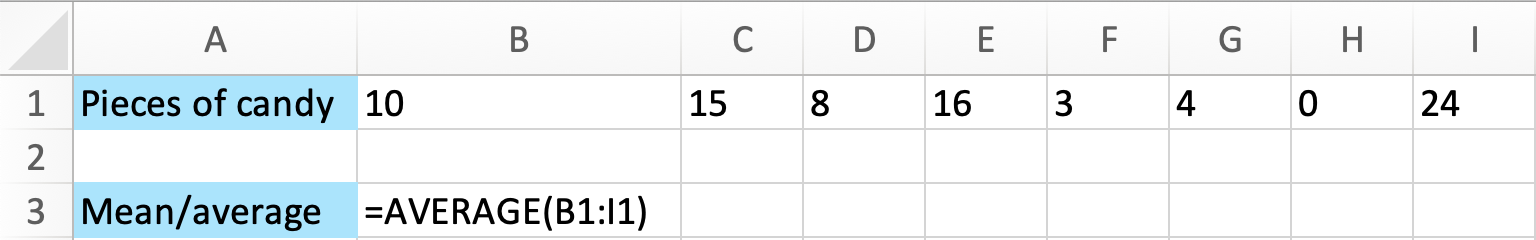# How to Calculate Average or Mean in Excel

Calculating the mean, or average, of a data set is actually pretty easy. You just add all the values of the data, and then divide that sum by the number of data points. This can be a challenging task if the data consists of a large amount of numbers. In those cases you can use `Excel` to do the job for you.

`Excel` Instruction

### CalculatingtheMean

1.
Input the data in `Excel`.
2.
Click a nearby empty cell where you want the mean value to be shown. Write

=AVERAGE(

3.
Then mark all the numbers of the data and press `Enter`.

Example 1

Below, you can see an overview of how many pieces of candy eight of the students in a class ate this weekend:

 $10,15,8,16,3,4,0,24$

Find the mean of this data.

1.
First you enter all the data values in `Excel`, into individual cells.
2.
Then you enter the formula for finding the mean in an empty cell. Write

=AVERAGE(

3.
Then you highlight the cell range containing your data and press `Enter`. Simple as that!The result is:From `Excel` you see that the mean amount of candy eaten this weekend is 10 pieces.